Algebraic Loop Concepts

In a Simulink® model, an algebraic loop occurs when a signal loop exists with only direct feedthrough blocks within the loop. Direct feedthrough means that Simulink needs the value of the block’s input signal to compute its output at the current time step. Such a signal loop creates a circular dependency of block outputs and inputs in the same time-step. This results in an algebraic equation that needs solving at each time-step, adding computational cost to the simulation.

Some examples of blocks with direct feedthrough inputs are:

Nondirect feedthrough blocks maintain a State variable. Two examples are Integrator and Unit Delay.

Tip

To determine if a block has direct feedthrough, read the Characteristics section of the block reference page.

The figure shows an example of an algebraic loop. The Sum block is an algebraic variable xa that is constrained to equal the first input u minus xa (for example, xa = uxa).The solution of this simple loop is xa = u/2.

Mathematical Interpretation

Simulink contains a suite of numerical solvers for simulating ordinary differential equations (ODEs), which are systems of equations that you can write as

$\stackrel{˙}{x}=f\left(x,t\right),$

where x is the state vector and t is the independent time variable.

Some systems of equations contain additional constraints that involve the independent variable and the state vector, but not the derivative of the state vector. Such systems are called differential algebraic equations (DAEs),

The term algebraic refers to equations that do not involve any derivatives. You can express DAEs that arise in engineering in the semi-explicit form

$\begin{array}{l}\stackrel{˙}{x}=f\left(x,{x}_{a},t\right)\\ 0=g\left(x,{x}_{a},t\right),\end{array}$

where:

• f and g can be vector functions.

• The first equation is the differential equation.

• The second equation is the algebraic equation.

• The vector of differential variables is x.

• The vector of algebraic variables is xa.

In Simulink models, algebraic loops are algebraic constraints. Models with algebraic loops define a system of differential algebraic equations. Simulink solves the algebraic equations (the algebraic loop) numerically for xa at each step of the ODE solver.

The model in the figure is equivalent to this system of equations in semi-explicit form:

$\begin{array}{l}\stackrel{˙}{x}=f\left(x,{x}_{a},t\right)={x}_{a}\\ 0=g\left(x,{x}_{a},t\right)=-x+u-2{x}_{a}.\end{array}$At each step of the ODE solver, the algebraic loop solver must solve the algebraic constraint for xa before calculating the derivative $\stackrel{˙}{x}$.

Physical Interpretation

Algebraic constraints:

• Occur when modeling physical systems, often due to conservation laws, such as conservation of mass and energy

• Occur when you choose a particular coordinate system for a model

• Help impose design constraints on system responses in a dynamic system

Use Simscape™ to model systems that span mechanical, electrical, hydraulic, and other physical domains as physical networks. Simscape constructs the DAEs that characterize the behavior of a model. The software integrates these equations with the rest of the model and then solves the DAEs directly. Simulink solves the variables for the components in the different physical domains simultaneously, avoiding problems with algebraic loops.

Artificial Algebraic Loops

An artificial algebraic loop occurs when an atomic subsystem or Model block causes Simulink to detect an algebraic loop, even though the contents of the subsystem do not contain a direct feedthrough from the input to the output. When you create an atomic subsystem, all Inport blocks are direct feedthrough, resulting in an algebraic loop.

Start with the included model, which represents a simple proportional control of the plant described by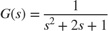which can be rewritten in state-space form as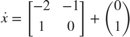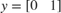The system has neither algebraic variables nor direct feedthrough and does not contain an algebraic loop.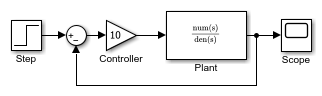Modify the model as described in the following steps:

1. Enclose the Controller and Plant blocks in a subsystem.

2. In the subsystem dialog box, select Treat as atomic unit to make the subsystem atomic.

3. In the Diagnostics pane of the Model Configuration Parameters, set the Algebraic Loop parameter to error.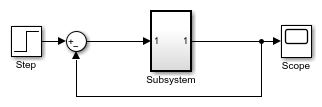When simulating this model, an algebraic loop occurs because the subsystem is direct feedthrough, even though the path within the atomic subsystem is not direct feedthrough. Simulation stops with an algebraic loop error.

How the Algebraic Loop Solver Works

When a model contains an algebraic loop, Simulink uses a nonlinear solver at each time step to solve the algebraic loop. The solver performs iterations to determine the solution to the algebraic constraint, if there is one. As a result, models with algebraic loops can run more slowly than models without algebraic loops.

Simulink uses a dogleg trust region algorithm to solve algebraic loops. The tolerance used is smaller than the ODE solver Reltol and Abstol. This is because Simulink uses the “explicit ODE method” to solve Index-1 differential algebraic equations (DAEs).

For the algebraic loop solver to work,

• There must be one block where the loop solver can break the loop and attempt to solve the loop.

• The model should have real double signals.

• The underlying algebraic constraint must be a smooth function

For example, suppose your model has a Sum block with two inputs—one additive, the other subtractive. If you feed the output of the Sum block to one of the inputs, you create an algebraic loop where all of the blocks include direct feedthrough.The Sum block cannot compute the output without knowing the input. Simulink detects the algebraic loop, and the algebraic loop solver solves the loop using an iterative loop. In the Sum block example, the software computes the correct result this way:

 xa(t) = u(t) / 2. (1)

The algebraic loop solver uses a gradient-based search method, which requires continuous first derivatives of the algebraic constraint that correspond to the algebraic loop. As a result, if the algebraic loop contains discontinuities, the algebraic loop solver can fail.

Trust-Region and Line-Search Algorithms in the Algebraic Loop Solver

The Simulink algebraic loop solver uses one of two algorithms to solve algebraic loops:

• Trust-Region

• Line-Search

By default, Simulink chooses the best algebraic loop solver and may switch between the two methods during simulation. To explicitly enable automatic algebraic loop solver selection for your model, at the MATLAB® command line, enter:

set_param(model_name, 'AlgebraicLoopSolver','Auto');

To switch to the trust-region algorithm, at the MATLAB command line, enter:

set_param(model_name, 'AlgebraicLoopSolver', 'TrustRegion');

If the algebraic loop solver cannot solve the algebraic loop with the trust-region algorithm, try simulating the model using the line-search algorithm.

To switch to the line-search algorithm, at the MATLAB command line, enter:

set_param(model_name, 'AlgebraicLoopSolver', 'LineSearch');

Limitations of the Algebraic Loop Solver

Algebraic loop solving is an iterative process. The Simulink algebraic loop solver is successful only if the algebraic loop converges to a definite answer. When the loop fails to converge, or converges too slowly, the simulation exits with an error.

The algebraic loop solver cannot solve algebraic loops that contain any of the following:

• Blocks with discrete-valued outputs

• Blocks with nondouble or complex outputs

• Discontinuities

• Stateflow® charts

Implications of Algebraic Loops in a Model

If your model contains an algebraic loop:

• You cannot generate code for the model.

• The Simulink algebraic loop solver might not be able to solve the algebraic loop.

• While Simulink is trying to solve the algebraic loop, the simulation can execute slowly.

For most models, the algebraic loop solver is computationally expensive for the first time step. Simulink solves subsequent time steps rapidly because a good starting point for xa is available from the previous time step.